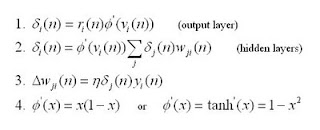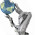## Saturday, April 16, 2011

### Neural Networks with C# - part 2

#### A High Level Understanding of Backpropagation Learning

Backpropagation is a technique used to help a feedforward neural networks learn or improve the accuracy of it's output based on the expected output of the learning sample set. That is to say, we will have a set of sample inputs with their expected outputs and then use that set to make adjustments in for the weights of our neuron associations (again I like to call them axons). This approach effectively solves the problem of how to update hidden layers.

#### The Math Basics for Backpropagation Learning1. ri is the error for the nth node of the ith layer. Furthermore, r is only for the output layer; it is simply the difference between the expected output and the output (see Neuron.CalcDelta() in example download). vi is the values calculated during the normal feedforward algorithms (see part 1). Φ' will be explained in equation 4. δi is the calculated change for neuron n within the ith layer.
2. Critical: This is much like equation 1 with some very distinct differences. You may notice that instead of using r, the error is calculated by the sum of the jth delta with the weighted association axons of the nth neuron with the jth layer.
3. This equation is the gradient or calculated change of weights. Or in other words, this is where we make the learned changes. η is simply a learning rate between 0 and 1. y is the original input values calculated from the axon for the nth neuron during the feedforward calculation.
4. This is the inverse or derivative activation function for either sigmoid or hyperbolic tangent functions (depends on whichever activation function was used for the neurons).
As mentioned in part 1, n is referring to the nth node in a series of node calculations from input to output. Also, i and j simply note the distinction between one layer and another.

<< back to part 1

1.hei,

I'm tring to understand the semple.
in NN.Models
the InverseActi.. is a function of V_i or of Y_i?

2.is about Neuron class

3.Hello.
I'm working on my PhD and found your sample really great, clear, object oriented.
On top of that, it is very automnous ans that's what I really need. Do you allow me to fork your project in a open-source gitHub project ? I could be more precise in a full mail, contat me : contact[at]cosmogonies.net I'm doing R&D into MotionCapture Animation augmented with NN.

4.I feel that you simply could do with
a few p.c. to pressure the message home a little bit, but instead of that, this is excellent blog.
A great read. I'll definitely be back.